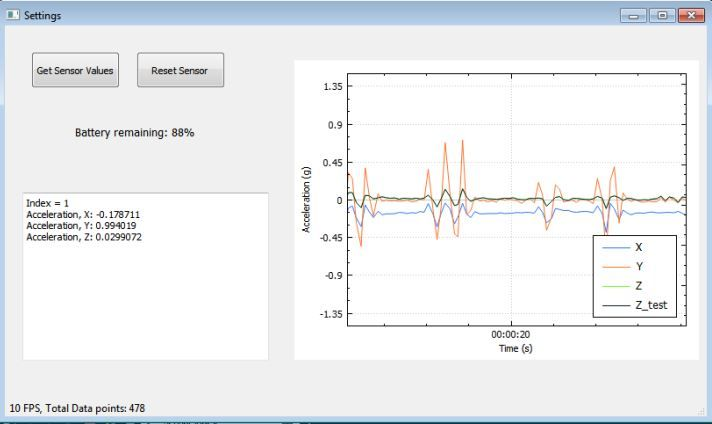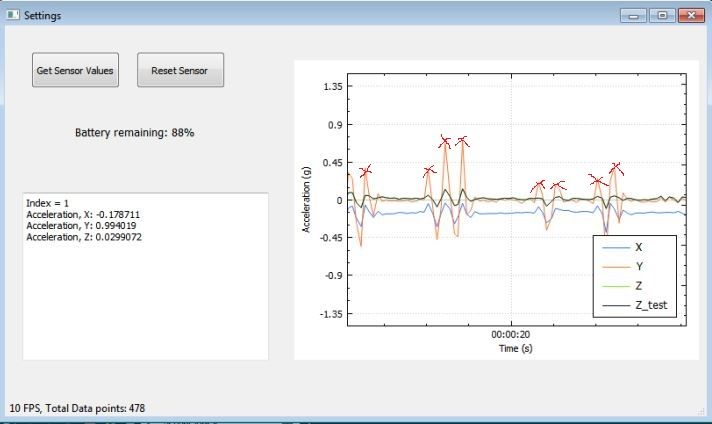# Detecting peak value on realtime data stream

• Hi all,

This is a bit off topic question! but hope I get some feedback from this forum.

I'm using QCustomPlot to display realtime value from an IMU.

This is how I set the `realtimeDataSlot`:

``````void Settings::realtimeDataSlot(double x_acceleration_g, double y_acceleration_g, double z_acceleration_g, double z_acceleration_gnew)
{
static QTime time(QTime::currentTime());
// calculate two new data points:
double key = time.elapsed()/1000.0; // time elapsed since start of demo, in seconds
static double lastPointKey = 0;
if (key-lastPointKey > 0.02) // at most add point every 20 ms
{

lastPointKey = key;
}
// make key axis range scroll with the data (at a constant range size of 8):
ui->customPlot->xAxis->setRange(key, 8, Qt::AlignRight);
ui->customPlot->replot();

// calculate frames per second:
static double lastFpsKey;
static int frameCount;
++frameCount;
if (key-lastFpsKey >2) // average fps over 2 seconds
{
ui->statusbar->showMessage(
QString("%1 FPS, Total Data points: %2")
.arg(frameCount/(key-lastFpsKey), 0, 'f', 0)
.arg(ui->customPlot->graph(0)->data()->size()+ui->customPlot->graph(1)->data()->size())
, 0);
lastFpsKey = key;
frameCount = 0;
}
}
``````

which shows me as follows:As a next step, I need to detect the peaks (I define peak as that value (positive values) more than 0.25 g at high rate.) in any axis, say for example in the above figure in the Y axis there are peak values which I need to detect and count. Can somebody show me a way to do this?

I need to mark peaks as follows:• I need to detect the peaks (I define peak as that value (positive values) more than 0.25 g at high rate.) in any axis, say for example in the above figure in the Y axis there are peak values which I need to detect and count. Can somebody show me a way to do this?

"Peak detection" is quite a big field.

You can start by reading up on peak detection algorithms, or using a peak detection library in your application: https://www.google.com/search?q=peak+detection+library+c%2B%2B